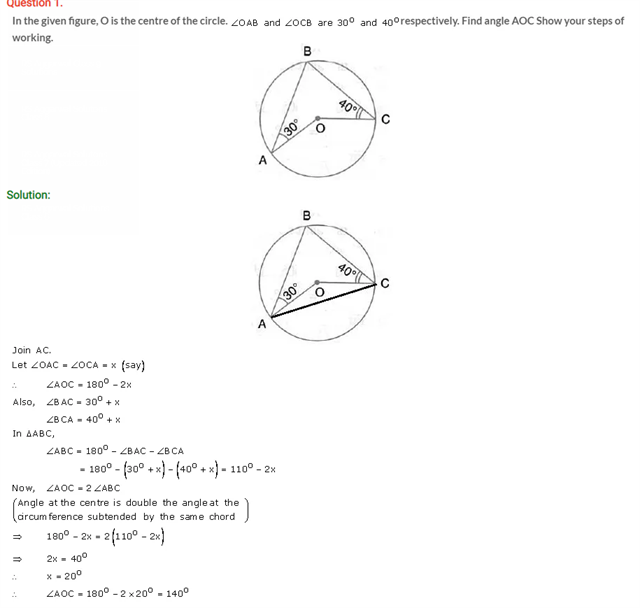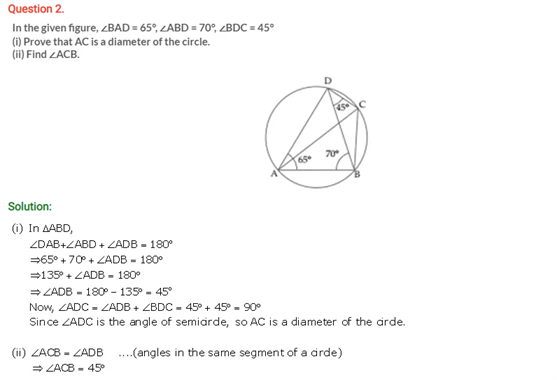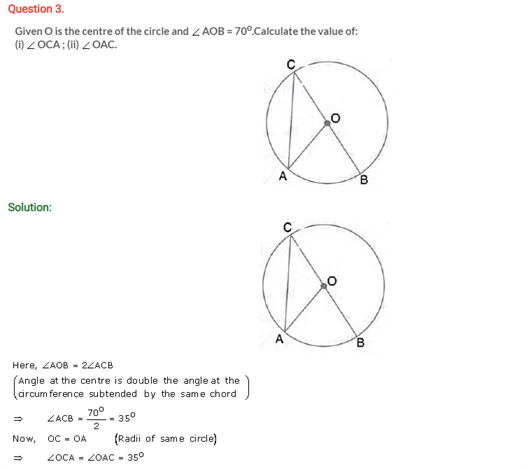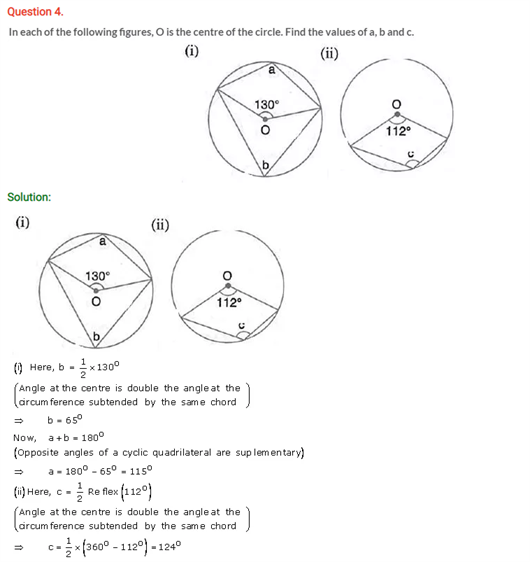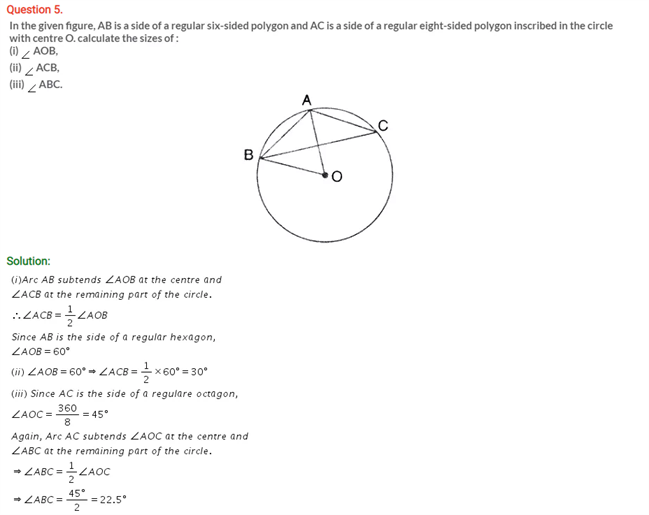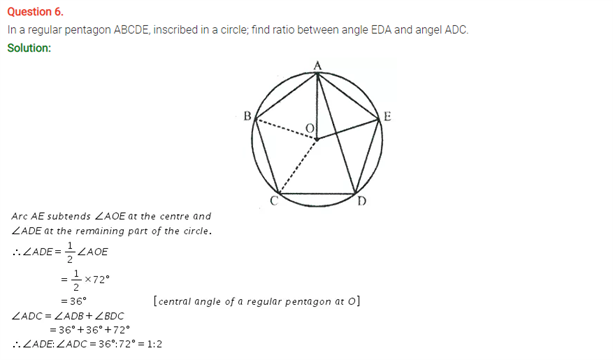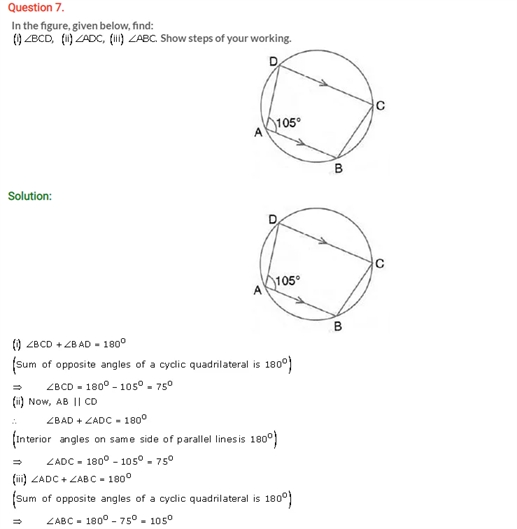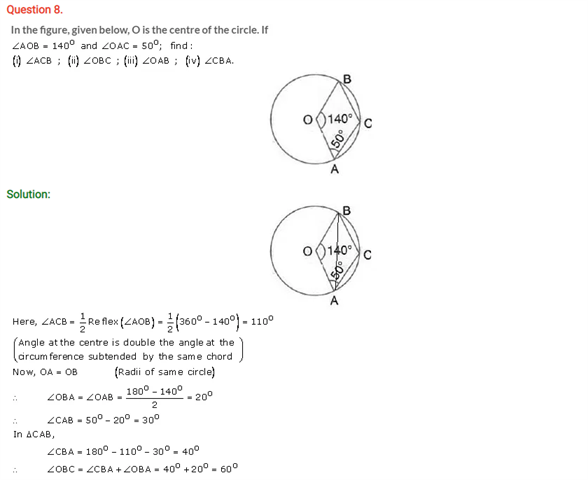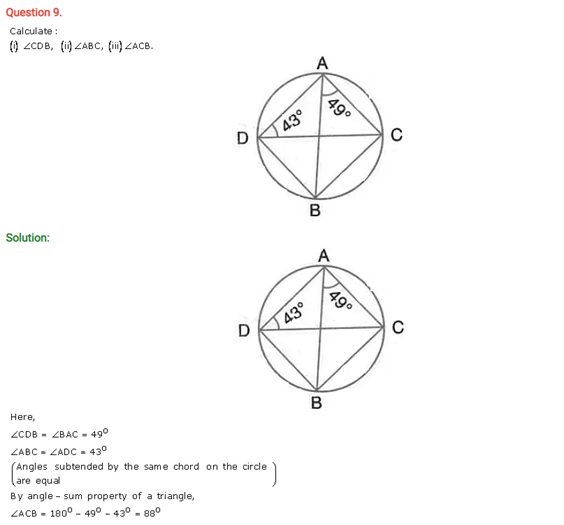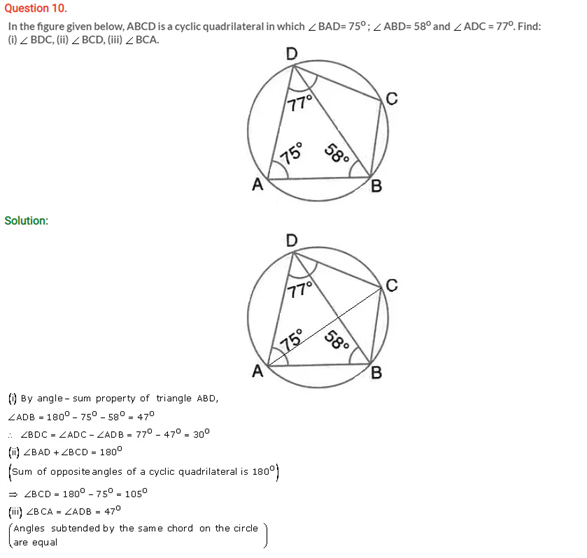# Selina Concise Maths Solution for ICSE Class 10 Chapter 17 Circles

## Selina Class 10 Maths Solutions for Chapter 17

A circle is a kind of line segment which is bent around until its ends join. Solving the solutions on circles and its properties from the ICSE Solutions For Class 10 Maths will ensure a better grasp of this chapter. A circle has many properties such as center is a point from which all the points are equidistant, a radius is the length from the center of the circle to any point on the circle, a secant is a line intersecting the circle at two points, a chord is a line segment connecting two points in a circle, a tangent is the line passing a circle and touching it at only one point. These questions below belong to the above concepts.

Students can view ICSE Class 10 Maths Selina Solutions Chapter 17 Circles below: